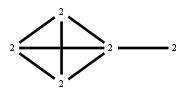# The Markov Bases Database

## G45g_bin

The binary graphical model of G45.

It is a hierarchical model of 5 variables. The dimension of the model is 17.The cardinality of the statespace is 32.

### Properties of the Markov basis

 Markov degree 2 56

 degree 2 56

The model has the following properties:

• All variables are binary.
• It is a graphical model.
• The minimal Markov basis is unique.
• The semigroup is normal.### FILES

 Markov basis: sufficient statistics matrix: G45g_bin.mar (5.31 kb) G45g_bin.mat (1.28 kb) G45g_bin.mod (32 b) G45g_bin.tar.gz (4.03 kb)